nextnano.com  nextnano³  Download | Search | Copyright | Publications  * password protected nextnano³ software1D pn-junction

# nextnano3 - Tutorial

## pn-junction

Author: Stefan Birner

```-> GaAs_pn_junction_1D_nn3.in             / *_nnp.in  - ```input file for the nextnano3 and nextnano++ software``` -> GaAs_pn_junction_1D_QM_nn3.in                      - ```input file for the nextnano3 software``` -> GaAs_pn_junction_2D_nn3.in             / *_nnp.in  - ```input file for the nextnano3 and nextnano++ software``` -> GaAs_pn_junction_3D_nn3.in             / *_nnp.in  - ```input file for the nextnano3 and nextnano++ software``` -> GaAs_pn_junction_1D_ForwardBias_nn3.in / *_nnp.in  - ```input file for the nextnano³ and nextnano++ software
```-> GaAs_pn_junction_2D_ForwardBias_nnp.in             - ```input file for the nextnano++ software``` -> GaAs_pn_junction_3D_ForwardBias_nnp.in             - ```input file for the nextnano++ software

## pn-junction

`-> GaAs_pn_junction_1D_nn3.in / *_nnp.in  - `input file for the nextnano3 and nextnano++ software

This tutorial aims to reproduce figure 3.1 (p. 51) of Joachim Piprek's book "Semiconductor Optoelectronic Devices - Introduction to Physics and Simulation" (Section 3.2 "pn-Junctions").

### Doping concentration

• The structure consists of 300 nm GaAs.
At the left and right boundaries, metal contacts are connected to the GaAs semiconductor (i.e. from 0 nm to 10 nm, and from 310 nm to 320 nm).
The structure is p-type doped from 10 nm to 160 nm and n-type doped from 160 nm to 310 nm.
• The following figure shows the concentration of donors and acceptors of the pn-junction.
In the p-type region between 10 nm and 160 nm, the number of acceptors NA is 0.5 x 1018 cm-3.
In the n-type region between 160 nm and 310 nm, the number of donors ND is 2.0 x 1018 cm-3.### Carrier concentrations

• The equilibrium condition for a pn-junction is achieved by a small transfer of electrons from the n region to the p region, where they recombine with holes. This leads to a depletion region (depletion width = wp + wn), i.e. the region around the pn-junction only has very few free carriers left.
• The following figure shows the electron and hole densities and the depletion region around the pn-junction at 160 nm. Here, we assumed that all donors and acceptors are fully ionized.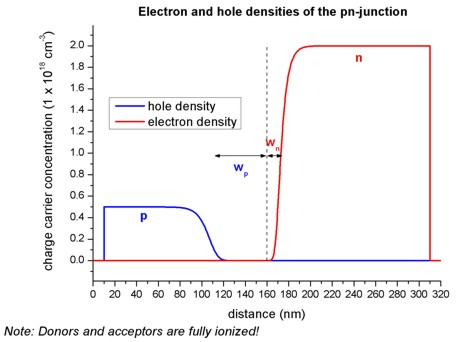### Net charges (space charge)

• In the depletion region, a net charge results from the ionized donors ND and ionized acceptors NA.
• The following figure shows the net charge density of the pn-junction.### Electric field

• The slope of the electric field is proportional to the net charge (Poisson equation), thus the extremum of the electric field is expected to be at the pn-junction.
• In regions without charges, the electric field is zero.
• The following figure shows the electric field of the pn-junction.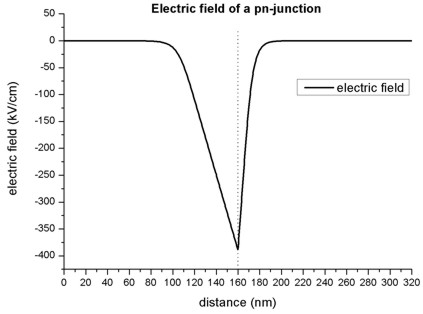The extremum of the electric field Fmax (at 160 nm) can be approximated as follows:
Fmax = `-` e NA wp / (epsilon epsilon0) = `-` 6.997 x 1014 V/m2 wp = 387 kV/cm
= `-` e ND wn / (epsilon epsilon0) = `-` 2.799 x 1015 V/m2 wn = 386 kV/cm

where
e = 1.6022 x 10-19 As
epsilon = 12.93 (dielectric constant of GaAs)
epsilon0 = 8.854 x 10-12 As/(Vm)
NA = 0.5 x 1018 cm-3
ND = 2.0 x 1018 cm-3
wp = 55.3 nm
wn = 13.8 nm

### Electrostatic potential, conduction and valence band edges

• In regions, where the electric field is zero, the electrostatic potential is constant.
• The electrostatic potential phi determines the conduction and valence band edges:
Ec = Ec0 `-` e phi
Ev = Ev0 `-` e phi
• The following figure shows the conduction and valence band edges, the electrostatic potential and the Fermi level of the pn-junction.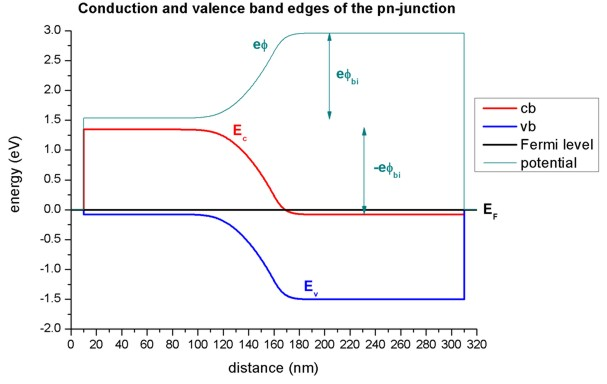Without external bias (i.e. equilibrium), the Fermi level EF is constant (EF = 0 eV).

The built-in potential phibi was calculated by nextnano³ to be equal to 1.426 V.
It can be approximated as follows:
phibi = Fmax (wp + wn) / 2
Assuming Fmax = 387 kV/cm, this would indicate for the depletion width: wp + wn = 73.7 nm.

To allow for a constant chemical potential (i.e. constant Fermi level EF), a total potential difference of -e phibi is required.

### Quantum mechanical calculation

`-> GaAs_pn_junction_1D_QM_nn3.in`

• Here, instead of calculating the densities classically, we solve the Schroedinger equation for the electrons, light and heavy holes in the single-band approximation over the whole device. We calculate up to 300 eigenvalues for each band. Thus the electron and hole densities are calculated purely quantum mechanically.
• The following figure shows the electron and hole concentrations for the classical and quantum mechanical calculations. For the QM calculations, different boundary conditions were used.
- Dirichlet boundary conditions force the wave functions to be zero at the boundaries, thus the density goes to zero at the boundaries which is unphysically.
- Neumann boundary conditions lead to unphysically large values at the boundaries.
- Mixed boundary conditions are in between. (This feature is not supported any more.)
For the classical calculation, the densities at the boundaries are constant.
Nevertheless, in the interesting region around the pn-junction, all four options lead to identical densities.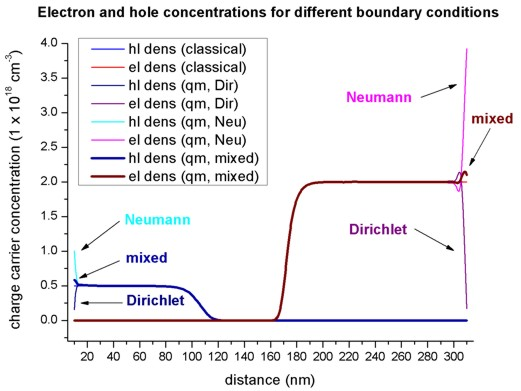• The following figure shows the band edges of the pn-junction for the four cases:
- classical calculation
- quantum mechanical calculation with Dirichlet boundary conditions
- quantum mechanical calculation with Neumann boundary conditions
- quantum mechanical calculation with mixed boundary conditions (This feature is not supported any more.)
For all cases the band edges are identical in the area around the pn-junction. Tiny deviations exist at the boundaries of the device.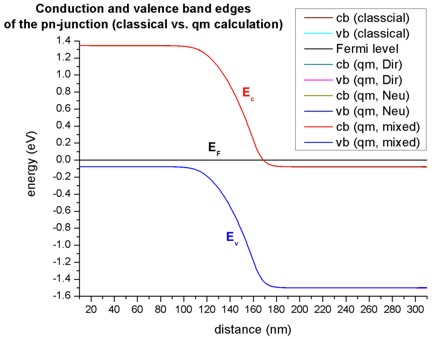• This figure is a zoom into the right boundary of the conduction band edge.
On this scale, the tiny deviations for the different boundary conditions can be clearly seen.### Nonequilibrium

• So-called "quasi-Fermi levels" which are different for electrons (EF,n) and holes (EF,p) are used to describe nonequilibrium carrier concentrations.
In equilibrium the quasi-Fermi levels are constant and have the same value for both electrons and holes (EF,n = EF,p = 0 eV).
The current is proportional to the mobility and the gradient of the quasi-Fermi level EF.

```-> GaAs_pn_junction_1D_ForwardBias_nn3.in / _nnp.in - ```input file for the nextnano³ software and nextnano++ software

### 2D/3D simulations

`-> GaAs_pn_junction_2D_nn3.in / *_nnp.in  - `input file for the nextnano3 and nextnano++ software``` -> GaAs_pn_junction_3D_nn3.in / *_nnp.in  - ```input file for the nextnano3 and nextnano++ software

Input files for the same pn junction structure as in 1D, but this time for a 2D and 3D simulation are also available.
==> 2D: rectangle of dimension 320 nm x 200 nm
==> 3D: cuboid     of dimension 320 nm x 200 nm x 100 nm